The INTPOINT Procedure
 Mathematical Description of LP

A linear programming (LP) problem has a linear objective function and a collection of linear constraints. PROC INTPOINT finds the values of variables that minimize the total cost of the solution. The value of each variable is on or between the variable’s lower and upper bounds, and the constraints are satisfied.

If an LP hasvariables andconstraints, then the formal statement of the problem solved by PROC INTPOINT is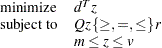where

•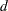is the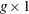variable objective function coefficient vector

•is thevariable value vector

•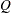is the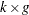constraint coefficient matrix for the variables, where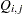is the coefficient of variablein theth constraint

•is the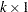side constraint right-hand-side vector

•is thevariable lower bound vector

•is thevariable upper bound vectorPrevious Page | Next Page | Top of Page